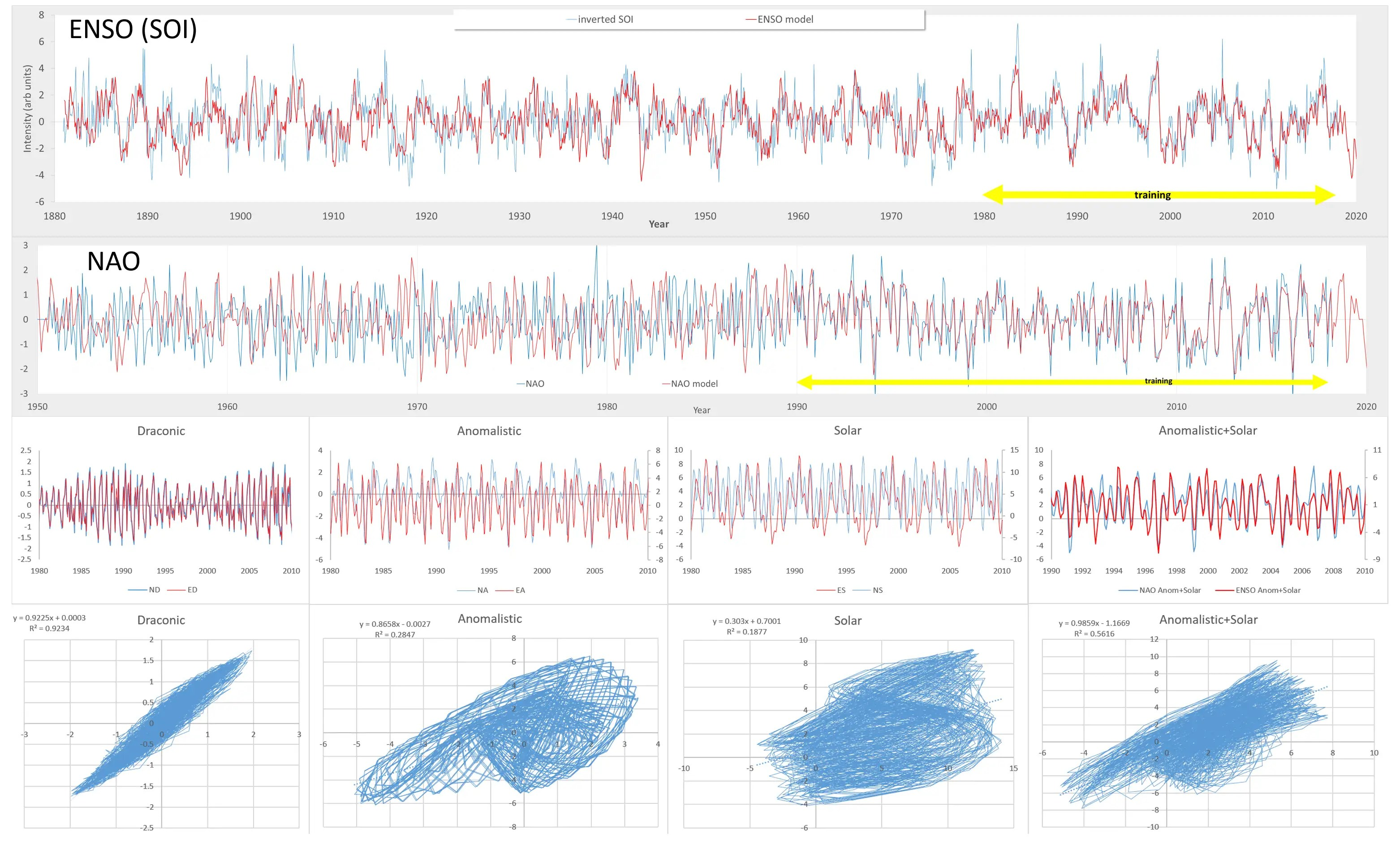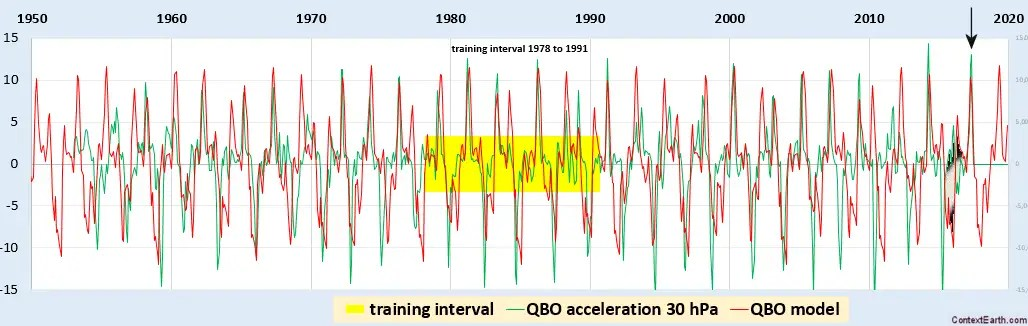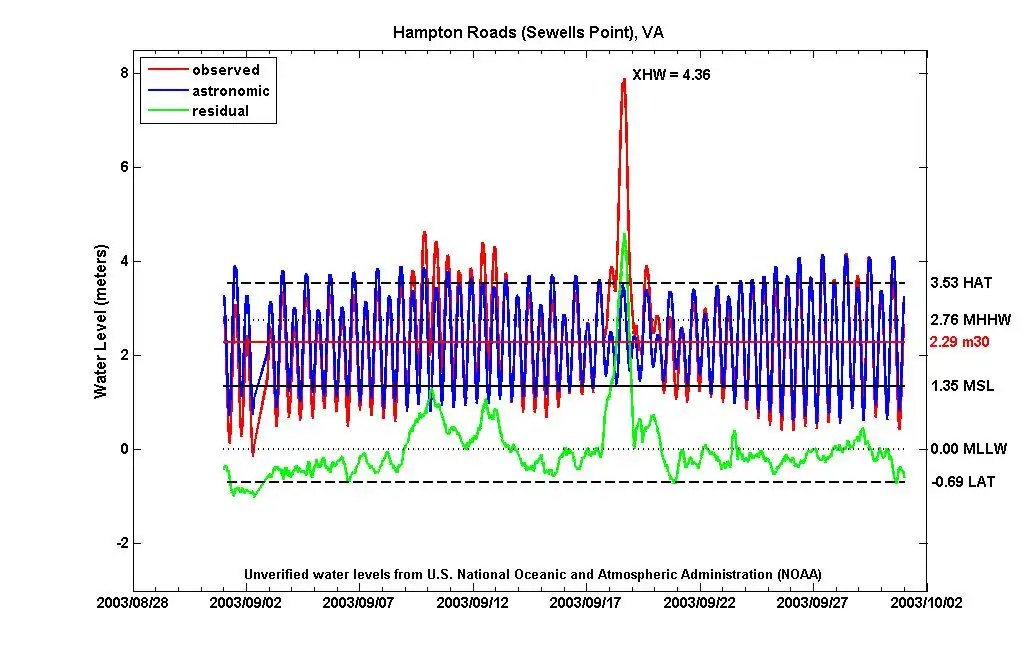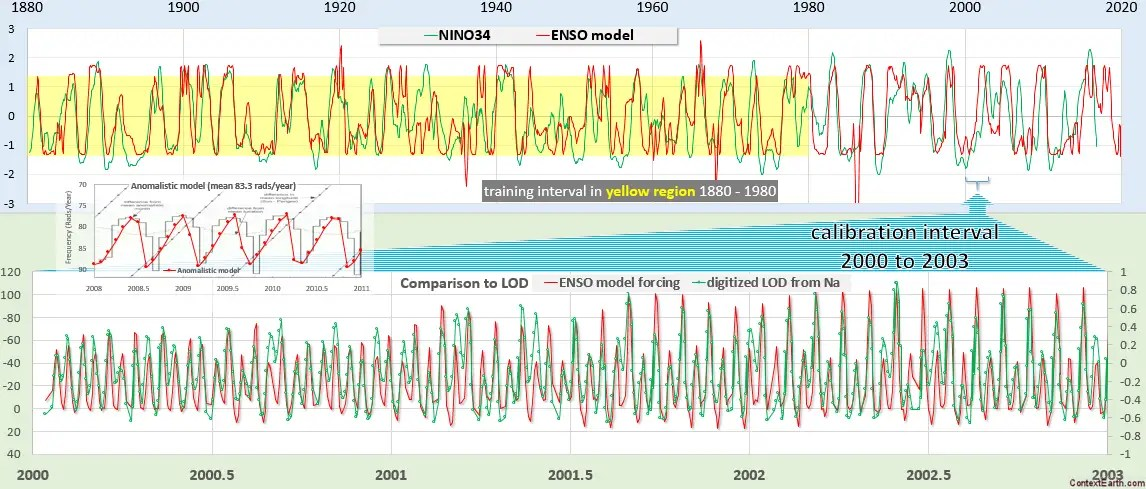# The SAO and Annual Disturbances

In Chapter 11 of the book Mathematical GeoEnergy, we model the QBO of equatorial stratospheric winds, but only touch on the related cycle at even higher altitudes, the semi-annual oscillation (SAO). The figure at the top of a recent post geometrically explains the difference between SAO and QBO — the basic idea is that the SAO follows the solar tide and not the lunar tide because of a lower atmospheric density at higher altitudes. Thus, the heat-based solar tide overrides the gravitational lunar+solar tide and the resulting oscillation is primarily a harmonic of the annual cycle.Figure 1 : The SAO modeled with the GEM software fit to 1 hPa data along the equator Continue reading

# Mathematical Geoenergy

Our book Mathematical Geoenergy presents a number of novel approaches that each deserve a research paper on their own. Here is the list, ordered roughly by importance (IMHO):

1. Laplace’s Tidal Equation Analytic Solution.
(Ch 11, 12) A solution of a Navier-Stokes variant along the equator. Laplace’s Tidal Equations are a simplified version of Navier-Stokes and the equatorial topology allows an exact closed-form analytic solution. This could classify for the Clay Institute Millenium Prize if the practical implications are considered, but it’s a lower-dimensional solution than a complete 3-D Navier-Stokes formulation requires.
2. Model of El Nino/Southern Oscillation (ENSO).
(Ch 12) A tidally forced model of the equatorial Pacific’s thermocline sloshing (the ENSO dipole) which assumes a strong annual interaction. Not surprisingly this uses the Laplace’s Tidal Equation solution described above, otherwise the tidal pattern connection would have been discovered long ago.
3. Model of Quasi-Biennial Oscillation (QBO).
(Ch 11) A model of the equatorial stratospheric winds which cycle by reversing direction ~28 months. This incorporates the idea of amplified cycling of the sun and moon nodal declination pattern on the atmosphere’s tidal response.
4. Origin of the Chandler Wobble.
(Ch 13) An explanation for the ~433 day cycle of the Earth’s Chandler wobble. Finding this is a fairly obvious consequence of modeling the QBO.
5. The Oil Shock Model.
(Ch 5) A data flow model of oil extraction and production which allows for perturbations. We are seeing this in action with the recession caused by oil supply perturbations due to the Corona Virus pandemic.
6. The Dispersive Discovery Model.
(Ch 4) A probabilistic model of resource discovery which accounts for technological advancement and a finite search volume.
7. Ornstein-Uhlenbeck Diffusion Model
(Ch 6) Applying Ornstein-Uhlenbeck diffusion to describe the decline and asymptotic limiting flow from volumes such as occur in fracked shale oil reservoirs.
8. The Reservoir Size Dispersive Aggregation Model.
(Ch 4) A first-principles model that explains and describes the size distribution of oil reservoirs and fields around the world.
9. Origin of Tropical Instability Waves (TIW).
(Ch 12) As the ENSO model was developed, a higher harmonic component was found which matches TIW
10. Characterization of Battery Charging and Discharging.
(Ch 18) Simplified expressions for modeling Li-ion battery charging and discharging profiles by applying dispersion on the diffusion equation, which reflects the disorder within the ion matrix.
11. Anomalous Behavior in Dispersive Transport explained.
(Ch 18) Photovoltaic (PV) material made from disordered and amorphous semiconductor material shows poor photoresponse characteristics. Solution to simple entropic dispersion relations or the more general Fokker-Planck leads to good agreement with the data over orders of magnitude in current and response times.
12. Framework for understanding Breakthrough Curves and Solute Transport in Porous Materials.
(Ch 20) The same disordered Fokker-Planck construction explains the dispersive transport of solute in groundwater or liquids flowing in porous materials.
13. Wind Energy Analysis.
(Ch 11) Universality of wind energy probability distribution by applying maximum entropy to the mean energy observed. Data from Canada and Germany. Found a universal BesselK distribution which improves on the conventional Rayleigh distribution.
14. Terrain Slope Distribution Analysis.
(Ch 16) Explanation and derivation of the topographic slope distribution across the USA. This uses mean energy and maximum entropy principle.
15. Thermal Entropic Dispersion Analysis.
(Ch 14) Solving the Fokker-Planck equation or Fourier’s Law for thermal diffusion in a disordered environment. A subtle effect but the result is a simplified expression not involving complex errf transcendental functions. Useful in ocean heat content (OHC) studies.
16. The Maximum Entropy Principle and the Entropic Dispersion Framework.
(Ch 10) The generalized math framework applied to many models of disorder, natural or man-made. Explains the origin of the entroplet.
17. Solving the Reserve Growth “enigma”.
(Ch 6) An application of dispersive discovery on a localized level which models the hyperbolic reserve growth characteristics observed.
18. Shocklets.
(Ch 7) A kernel approach to characterizing production from individual oil fields.
19. Reserve Growth, Creaming Curve, and Size Distribution Linearization.
(Ch 6) An obvious linearization of this family of curves, related to Hubbert Linearization but more useful since it stems from first principles.
20. The Hubbert Peak Logistic Curve explained.
(Ch 7) The Logistic curve is trivially explained by dispersive discovery with exponential technology advancement.
21. Laplace Transform Analysis of Dispersive Discovery.
(Ch 7) Dispersion curves are solved by looking up the Laplace transform of the spatial uncertainty profile.
22. Gompertz Decline Model.
(Ch 7) Exponentially increasing extraction rates lead to steep production decline.
23. The Dynamics of Atmospheric CO2 buildup and Extrapolation.
(Ch 9) Convolving a fat-tailed CO2 residence time impulse response function with a fossil-fuel emissions stimulus. This shows the long latency of CO2 buildup very straightforwardly.
24. Reliability Analysis and Understanding the “Bathtub Curve”.
(Ch 19) Using a dispersion in failure rates to generate the characteristic bathtub curves of failure occurrences in parts and components.
25. The Overshoot Point (TOP) and the Oil Production Plateau.
(Ch 8) How increases in extraction rate can maintain production levels.
26. Lake Size Distribution.
(Ch 15) Analogous to explaining reservoir size distribution, uses similar arguments to derive the distribution of freshwater lake sizes. This provides a good feel for how often super-giant reservoirs and Great Lakes occur (by comparison).
27. The Quandary of Infinite Reserves due to Fat-Tail Statistics.
(Ch 9) Demonstrated that even infinite reserves can lead to limited resource production in the face of maximum extraction constraints.
28. Oil Recovery Factor Model.
(Ch 6) A model of oil recovery which takes into account reservoir size.
29. Network Transit Time Statistics.
(Ch 21) Dispersion in TCP/IP transport rates leads to the measured fat-tails in round-trip time statistics on loaded networks.
30. Particle and Crystal Growth Statistics.
(Ch 20) Detailed model of ice crystal size distribution in high-altitude cirrus clouds.
31. Rainfall Amount Dispersion.
(Ch 15) Explanation of rainfall variation based on dispersion in rate of cloud build-up along with dispersion in critical size.
32. Earthquake Magnitude Distribution.
(Ch 13) Distribution of earthquake magnitudes based on dispersion of energy buildup and critical threshold.
33. IceBox Earth Setpoint Calculation.
(Ch 17) Simple model for determining the earth’s setpoint temperature extremes — current and low-CO2 icebox earth.
34. Global Temperature Multiple Linear Regression Model
(Ch 17) The global surface temperature records show variability that is largely due to the GHG rise along with fluctuating changes due to ocean dipoles such as ENSO (via the SOI measure and also AAM) and sporadic volcanic eruptions impacting the atmospheric aerosol concentrations.
35. GPS Acquisition Time Analysis.
(Ch 21) Engineering analysis of GPS cold-start acquisition times. Using Maximum Entropy in EMI clutter statistics.
36. 1/f Noise Model
(Ch 21) Deriving a random noise spectrum from maximum entropy statistics.
37. Stochastic Aquatic Waves
(Ch 12) Maximum Entropy Analysis of wave height distribution of surface gravity waves.
38. The Stochastic Model of Popcorn Popping.
(Appx C) The novel explanation of why popcorn popping follows the same bell-shaped curve of the Hubbert Peak in oil production. Can use this to model epidemics, etc.
39. Dispersion Analysis of Human Transportation Statistics.
(Appx C) Alternate take on the empirical distribution of travel times between geographical points. This uses a maximum entropy approximation to the mean speed and mean distance across all the data points.

# Asymptotic QBO Period

The modeled QBO cycle is directly related to the nodal (draconian) lunar cycle physically aliased against the annual cycle.  The empirical cycle period is best estimated by tracking the peak acceleration of the QBO velocity time-series, as this acceleration (1st derivative of the velocity) shows a sharp peak. This value should asymptotically approach a 2.368 year period over the long term.  Since the recent data from the main QBO repository provides an additional acceleration peak from the past month, now is as good a time as any to analyze the cumulative data.The new data-point provides a longer period which compensated for some recent shorter periods, such that the cumulative mean lies right on the asymptotic line. The jitter observed is explainable in terms of the model, as acceleration peaks are more prone to align close to an annual impulse. But the accumulated mean period is still aligned to the draconic aliasing with this annual impulse. As more data points come in over the coming decades, the mean should vary less and less from the asymptotic value.

The fit to QBO using all the data save for the last available data point is shown below.  Extrapolating beyond the green arrow, we should see an uptick according to the red waveform.Adding the recent data-point and the blue waveform does follow the model.There was a flurry of recent discussion on the QBO anomaly of 2016 (shown as a split peak above), which implied that perhaps the QBO would be permanently disrupted from it’s long-standing pattern. Instead, it may be a more plausible explanation that the QBO pattern was not simply wandering from it’s assumed perfectly cyclic path but instead is following a predictable but jittery track that is a combination of the (physically-aliased) annual impulse-synchronized Draconic cycle together with a sensitivity to variations in the draconic cycle itself. The latter calibration is shown below, based on NASA ephermeris.This is the QBO spectral decomposition, showing signal strength centered on the fundamental aliased Draconic value, both for the data and the set by the model.The main scientist, Prof. Richard Lindzen, behind the consensus QBO model has been recently introduced here as being “considered the most distinguished living climate scientist on the planet”.  In his presentation criticizing AGW science , Lindzen claimed that the climate oscillates due to a steady uniform force, much like a violin oscillates when the steady force of a bow is drawn across its strings.  An analogy perhaps better suited to reality is that the violin is being played like a drum. Resonance is more of a decoration to the beat itself.
Keith?

 Professor Richard Lindzen slammed conventional global warming thinking warming as ‘nonsense’ in a lecture for the Global Warming Policy Foundation on Monday. ‘An implausible conjecture backed by false evidence and repeated incessantly … is used to promote the overturn of industrial civilization,’ he said in London. — GWPF

# AO

The Arctic Oscillation (AO) dipole has behavior that is correlated to the North Atlantic Oscillation (NAO) dipole.   We can see this in two ways. First, and most straight-forwardly, the correlation coefficient between the AO and NAO time-series is above 0.6.

Secondly, we can use the model of the NAO from the last post and refit the parameters to the AO data (data also here), but spanning an orthogonal interval. Then we can compare the constituent lunisolar factors for NAO and AO for correlation, and further discover that this also doubles as an effective cross-validation for the underlying LTE model (as the intervals are orthogonal).Top panel is a model fit for AO between 1900-1950, and below that is a model fit for NAO between 1950-present. The lower pane is the correlation for a common interval (left) and for the constituent lunisolar factors for the orthogonal interval (right)

Only the anomalistic factor shows an imperfect correlation, and that remains quite high.

# NAO

The challenge of validating the models of climate oscillations such as ENSO and QBO, rests primarily in our inability to perform controlled experiments. Because of this shortcoming, we can either do (1) predictions of future behavior and validate via the wait-and-see process, or (2) creatively apply techniques such as cross-validation on currently available data. The first is a non-starter because it’s obviously pointless to wait decades for validation results to confirm a model, when it’s entirely possible to do something today via the second approach.

There are a variety of ways to perform model cross-validation on measured data.

In its original and conventional formulation, cross-validation works by checking one interval of time-series against another, typically by training on one interval and then validating on an orthogonal interval.

Another way to cross-validate is to compare two sets of time-series data collected on behaviors that are potentially related. For example, in the case of ocean tidal data that can be collected and compared across spatially separated geographic regions, the sea-level-height (SLH) time-series data will not necessarily be correlated, but the underlying lunar and solar forcing factors will be closely aligned give or take a phase factor. This is intuitively understandable since the two locations share a common-mode signal forcing due to the gravitational pull of the moon and sun, with the differences in response due to the geographic location and local spatial topology and boundary conditions. For tides, this is a consensus understanding and tidal prediction algorithms have stood the test of time.

In the previous post, cross-validation on distinct data sets was evaluated assuming common-mode lunisolar forcing. One cross-validation was done between the ENSO time-series and the AMO time-series. Another cross-validation was performed for ENSO against PDO. The underlying common-mode lunisolar forcings were highly correlated as shown in the featured figure.  The LTE spatial wave-number weightings were the primary discriminator for the model fit. This model is described in detail in the book Mathematical GeoEnergy to be published at the end of the year by Wiley.

Another common-mode cross-validation possible is between ENSO and QBO, but in this case it is primarily in the Draconic nodal lunar factor — the cyclic forcing that appears to govern the regular oscillations of QBO.  Below is the Draconic constituent comparison for QBO and the ENSO.The QBO and ENSO models only show a common-mode correlated response with respect to the Draconic forcing. The Draconic forcing drives the quasi-periodicity of the QBO cycles, as can be seen in the lower right panel, with a small training window.

This cross-correlation technique can be extended to what appears to be an extremely erratic measure, the North Atlantic Oscillation (NAO).NAO from NOAA https://www.ncdc.noaa.gov/teleconnections/nao/

Like the SOI measure for ENSO, the NAO is originally derived from a pressure dipole measured at two separate locations — but in this case north of the equator.  From the high-frequency of the oscillations, a good assumption is that the spatial wavenumber factors are much higher than is required to fit ENSO. And that was the case as evidenced by the figure below.ENSO vs NAO cross-validation

Both SOI and NAO are noisy time-series with the NAO appearing very noisy, yet the lunisolar constituent forcings are highly synchronized as shown by correlations in the lower pane. In particular, summing the Anomalistic and Solar constituent factors together improves the correlation markedly, which is because each of those has influence on the other via the lunar-solar mutual gravitational attraction. The iterative fitting process adjusts each of the factors independently, yet the net result compensates the counteracting amplitudes so the net common-mode factor is essentially the same for ENSO and NAO (see lower-right correlation labelled Anomalistic+Solar).

Since the NAO has high-frequency components, we can also perform a conventional cross-validation across orthogonal intervals. The validation interval below is for the years between 1960 and 1990, and even though the training intervals were aggressively over-fit, the correlation between the model and data is still visible in those 30 years.NAO model fit with validation spanning 1960 to 1990

Over the course of time spent modeling ENSO, the effort that went into fitting to NAO was a fraction of the original time. This is largely due to the fact that the temporal lunisolar forcing only needed to be tweaked to match other climate indices, and the iteration over the topological spatial factors quickly converges.

Many more cross-validation techniques are available for NAO, since there are different flavors of NAO indices available corresponding to different Atlantic locations, and spanning back to the 1800’s.

# Approximating the ENSO Forcing Potential

From the last post, we tried to estimate the lunar tidal forcing potential from the fitted harmonics of the ENSO model. Two observations resulted from that exercise: (1) the possibility of over-fitting to the expanded Taylor series, and (2) the potential of fitting to the ENSO data directly from the inverse power law.

The Taylor’s series of the forcing potential is a power-law polynomial corresponding to the lunar harmonic terms. The chief characteristic of the polynomial is the alternating sign for each successive power (see here), which has implications for convergence under certain regimes. What happens with the alternating sign is that each of the added harmonics will highly compensate the previous underlying harmonics, giving the impression that pulling one signal out will scramble the fit. This is conceptually no different than eliminating any one term from a sine or cosine Taylor’s series, which are also all compensating with alternating sign.

The specific conditions that we need to be concerned with respect to series convergence is when r (perturbations to the lunar orbit) is a substantial fraction of R (distance from earth to moon) :$F(r) = frac{1}{(R+r)^3}$

Because we need to keep those terms for high precision modeling, we also need to be wary of possible over-fitting of these terms — as the solver does not realize that the values for those terms have the constraint that they derive from the original Taylor’s series. It’s not really a problem for conventional tidal analysis, as the signals are so clean, but for the noisy ENSO time-series, this is an issue.

Of course the solution to this predicament is not to do the Taylor series harmonic fitting at all, but leave it in the form of the inverse power law. That makes a lot of sense — and the only reason for not doing this until now is probably due to the inertia of conventional wisdom, in that it wasn’t necessary for tidal analysis where harmonics work adequately.

So this alternate and more fundamental formulation is what we show here.

# Interface-Inflection Geophysics

This paper that a couple of people alerted me to is likely one of the most radical research findings that has been published in the climate science field for quite a while:

Topological origin of equatorial waves
Delplace, Pierre, J. B. Marston, and Antoine Venaille. Science (2017): eaan8819.

An earlier version on ARXIV was titled Topological Origin of Geophysical Waves, which is less targeted to the equator.

The scientific press releases are all interesting

What the science writers make of the research is clearly subjective and filtered through what they understand.

# The QBO anomaly of 2016 revisited

Remember the concern over the QBO anomaly/disruption during 2016?

Quite a few papers were written on the topic

1. Newman, P. A., et al. “The anomalous change in the QBO in 2015–2016.” Geophysical Research Letters 43.16 (2016): 8791-8797.
Newman, P. A., et al. “The Anomalous Change in the QBO in 2015-16.” AGU Fall Meeting Abstracts. 2016.
2. Randel, W. J., and M. Park. “Anomalous QBO Behavior in 2016 Observed in Tropical Stratospheric Temperatures and Ozone.” AGU Fall Meeting Abstracts. 2016.
3. Dunkerton, Timothy J. “The quasi‐biennial oscillation of 2015–2016: Hiccup or death spiral?.” Geophysical Research Letters 43.19 (2016).
4. Tweedy, O., et al. “Analysis of Trace Gases Response on the Anomalous Change in the QBO in 2015-2016.” AGU Fall Meeting Abstracts. 2016.
5. Osprey, Scott M., et al. “An unexpected disruption of the atmospheric quasi-biennial oscillation.” Science 353.6306 (2016): 1424-1427.
According to the lunar forcing model of QBO, which was also presented at AGU last year, the peak in acceleration should have occurred at the time pointed to by the BLACK downward arrow in the figure below. This was in April of this year. The GREEN is the QBO 30 hPa acceleration data and the RED is the QBO model.Note that the training region for the model is highlighted in YELLOW and is in the interval from 1978 to 1990. This was well in the past, yet it was able to pinpoint the sharp peak 27 years later.

The disruption in 2015-2016 shown with shaded black may have been a temporary forcing stimulus.  You can see that it obviously flipped the polarity with respect to the model. This will provoke a transient response in the DiffEq solution, which will then eventually die off.

The bottom-line is that the climate scientists who pointed out the anomaly were correct in that it was indeed a disruption, but this wasn’t necessarily because they understood why it occurred — but only that it didn’t fit a past pattern. It was good observational science, and so the papers were appropriate for publishing.  However, if you look at the QBO model against the data, you will see many similar temporary disruptions in the historical record. So it was definitely not some cataclysmic event as some had suggested. I think most scientists took a less hysterical view and simply pointed out the reversal in stratospheric winds was unusual.

I like to use this next figure as an example of how this may occur (found in the comment from last year). A local hurricane will temporarily impact the tidal displacement via a sea swell. You can see that in the middle of the trace below. On both sides of this spike, the tidal model is still in phase and so the stimulus is indeed transient while the underlying forcing remains invariant. For QBO, instead of a hurricane, the disruption could be caused by a SSW event. It also could be an unaccounted-for lunar forcing pulse not captured in the model. That’s probably worth more research.As the QBO is still on a 28 month alignment, that means that the external stimulus — as with ENSO, likely the lunar tidal force — is providing the boundary condition synchronization.

# Recipe for ENSO model in one tweetand for QBO